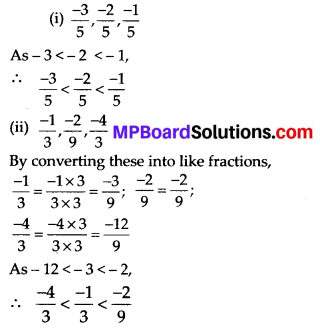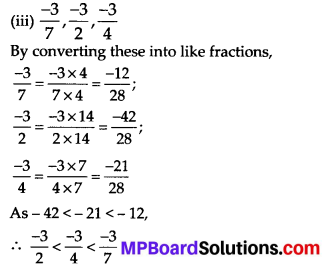# MP Board Class 7th Maths Solutions Chapter 9 Rational Numbers Ex 9.1

## MP Board Class 7th Maths Solutions Chapter 9 Rational Numbers Ex 9.1

Question 1.
List five rational numbers between:
(i) -1 and 0
(ii) -2 and -1
(iii) $$\frac{-4}{5}$$ and $$\frac{-2}{3}$$
(iv) $$\frac{1}{2}$$ and $$\frac{2}{3}$$
Solution:
(i) Five rational numbers between -1 and 0 are $$\frac{-1}{10}, \frac{-1}{20}, \frac{-1}{30}, \frac{-1}{40}, \frac{-1}{50}$$

(ii) -2 and -1
$$-2=\frac{-12}{6}$$ and $$-1=\frac{-6}{6}$$
∴ Five rational numbers between -2 and -1 are $$\frac{-11}{6}, \frac{-10}{6}, \frac{-9}{6}, \frac{-8}{6}, \frac{-7}{6}$$.

(iii)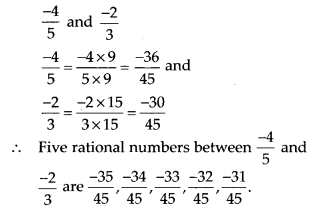(iv) $$\frac{1}{2}$$ and $$\frac{2}{3}$$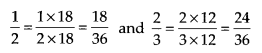∴ Five rational numbers between $$\frac{1}{2}$$ and $$\frac{2}{3}$$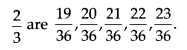Question 2.
Write four more rational numbers in each of the following patterns: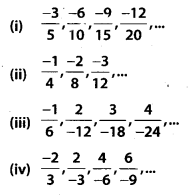Solution: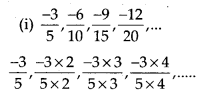Therefore, next four rational numbers in this pattern are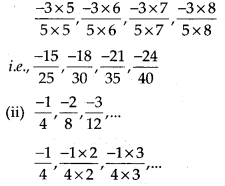Therefore, next four rational numbers in this pattern are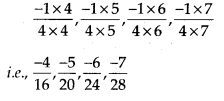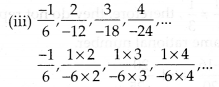Therefore, next four rational numbers in this pattern are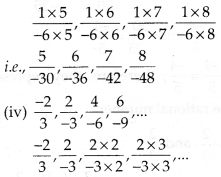Therefore, next four rational numbers in this pattern are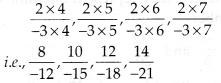Question 3.
Give four rational numbers equivalent to:
(i) $$\frac{-2}{7}$$
(ii) $$\frac{5}{-3}$$
(iii) $$\frac{4}{9}$$
Solution:
Four rational numbers are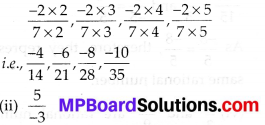Four rational numbers are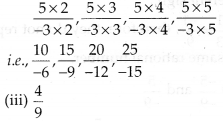Four rational numbers are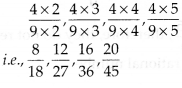Question 4.
Draw the number line and represent the following rational numbers on it:
(i) $$\frac{3}{4}$$
(ii) $$\frac{-5}{8}$$
(iii) $$\frac{-7}{4}$$
(iv) $$\frac{7}{8}$$
Solution:
(i) $$\frac{3}{4}$$
The fraction represents 3 parts out of 4 equal parts. Therefore, each space between two integers on the number line must be divided into 4 equal parts.
∴ $$\frac{3}{4}$$ can be represented as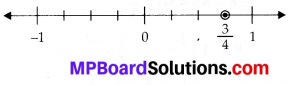(ii) $$\frac{-5}{8}$$
The fraction represents 5 parts out of 8 equal parts and negative sign represents that it is on the left side of the number line. Therefore, each space between two integers on the number line must be divided into 8 equal parts.
∴ $$\frac{5}{8}$$ can be represented as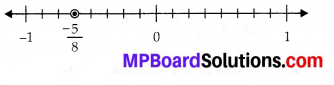(iii) $$\frac{-7}{4}$$ = $$-1 \frac{3}{4}$$
The fraction represents 1 full part and 3 parts out of 4 equal parts and negative sign represents that it is on the left side of the number line. Therefore, each space between two integers on the number line must be divided into 4 equal parts.
∴ $$\frac{-7}{4}$$ can be represented as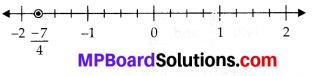(iv) $$\frac{7}{8}$$
The fraction represents 7 parts out of 8 equal parts. Therefore, each space between two integers on the number line must be divided into 8 equal parts.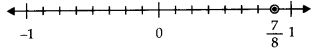Question 5.
The points P, Q, R, S, T, U, A and B on the number line are such that, TR = RS = SU and AP = PQ = QB. Name the rational numbers represented by P, Q, R and S.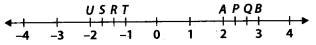Solution:
Distance between U and T = 1 unit
It is divided into 3 equal parts.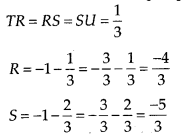Similarly, AB = 1 unit
It is divided into 3 equal parts.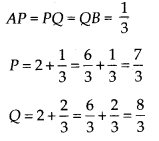Question 6.
Which of the following pairs represent the same rational number?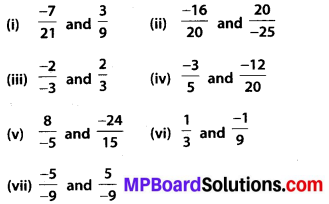Solution: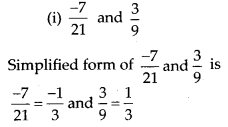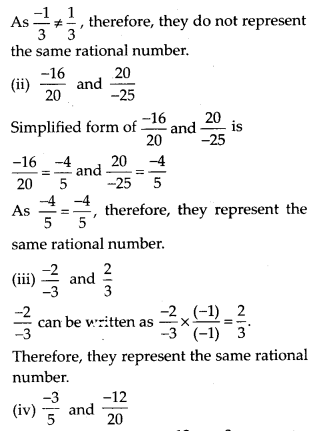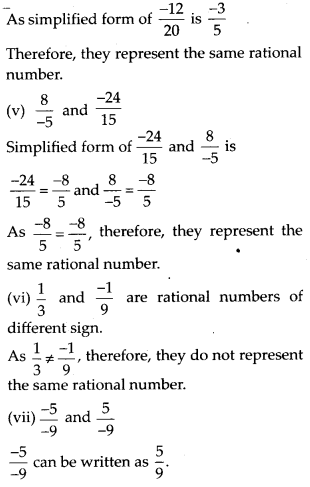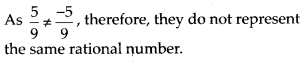Question 7.
Rewrite the following rational numbers in the simplest form: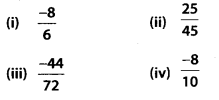Solution: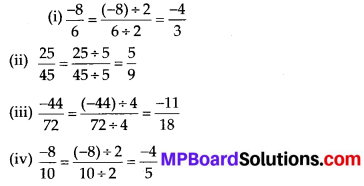Question 8.
Fill in the boxes with the correct symbol out of >, < and =.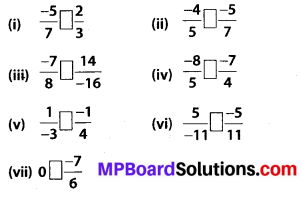Solution: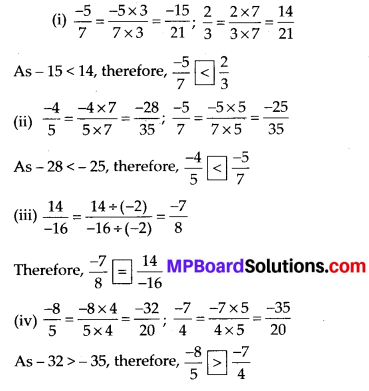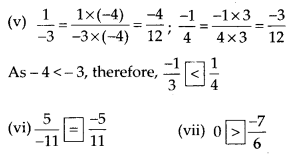Question 9.
Which is greater in each of the following: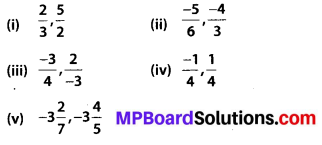Solution: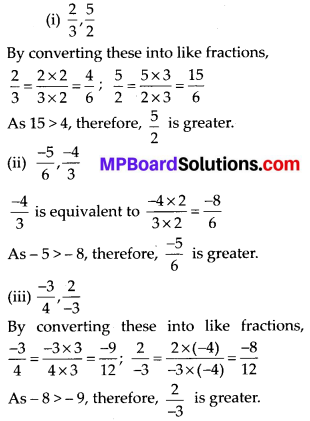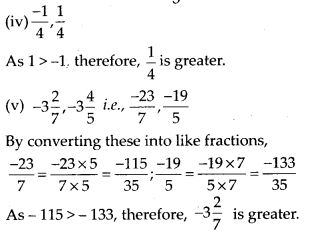Question 10.
Write the following rational numbers in ascending order: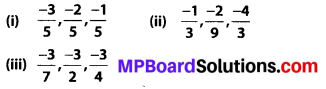Solution: